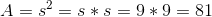# ISEE Lower Level Math : How to find the area of a square

## Example Questions

### Example Question #231 : Geometry

The perimeter of a square is 44 inches. What is the area?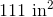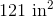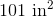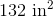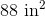Explanation:

In a square, all four sides are equal, and the area is calculated by multiplying one side by itself.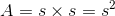To find the length of one side, we divide the perimeter by 4 (since there are 4 sides of the square).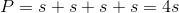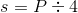We know the perimeter, allowing us to solve for the side.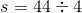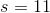This gives us 11, so we know that each side is 11 inches long. Now we can find the area.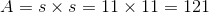The area, 11 inches times 11 inches, is 121 square inches, the correct answer.

### Example Question #12 : How To Find The Area Of A Square

A square has an area of 49 square inches. What is the length of one side of the square?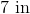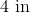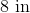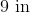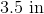Explanation:

In a square, all four sides are equal, and the area is calculated by multiplying one side by itself.Given that the area is 49 square inches, the length of one side of square would be 7, because 7 times 7 is 49.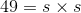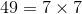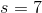### Example Question #13 : How To Find The Area Of A Square

If the perimeter of a square is 48 inches, how many square inches are in the area?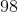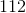Explanation:

The perimeter of a square is equal to the sum of the 4 sides. Given that all the sides of a square are equal, the length of one side of the square can be found by dividing the perimeter by 4.

Given that 48 divided by 4 is equal to 12, we can now find the area. The area is equal to one side multiplied by another. The result is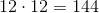### Example Question #14 : How To Find The Area Of A Square

If the perimeter of a square is 48 inches, what is the area?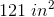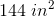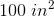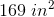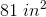Explanation:

The perimeter of a square is given by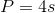, whereis the sidelength.

Plug in the given value for the perimeter: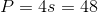Divide both sides by 4 to find the sidelength: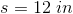The area of a square is given by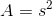Plug in the sidelength we just calculated to find the area: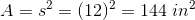### Example Question #15 : How To Find The Area Of A Square

If the perimeter of a square is 40 centimeters, what is the area?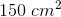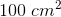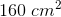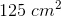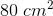Explanation:

The perimeter of a square is given by, whereis the sidelength.

We know the perimeter, so we can set up an equation to find the sidelength: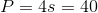Divide both sides by 4 to isolate the sidelength: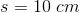The area of a square is given by.

We just calculated the sidelength, so now we can find the area: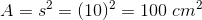### Example Question #16 : How To Find The Area Of A Square

The side of a square is 2 yards long. What is the area in square feet?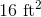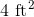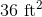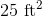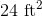Explanation:

To solve this question we will need to convert units from yards to feet.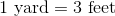Our question states that each side of the square is two yards.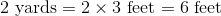Each side of the square is 6 feet. To find the area, we need to multiply the length of two sides.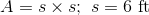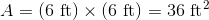### Example Question #17 : How To Find The Area Of A Square

If the perimeter of a square is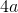, what is the area?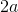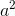Explanation:

If the perimeter of a square is, this means that each side is equal tobecause the side of a square is equal to one-fourth of its perimeter.

The area is the product of one side multiplied by another side.

Since all sides in a square are equal, the area would be equal tomultiplied by, which is: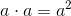### Example Question #18 : How To Find The Area Of A Square

What is the value of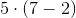?Explanation:simplies to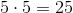. Therefore, 25 is the correct answer.

### Example Question #19 : How To Find The Area Of A Square

Find the area of a square whose side length is.Explanation:

To solve, simply use the formula for are of a square. Thus,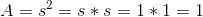### Example Question #20 : How To Find The Area Of A Square

Find the area of a square with side length.